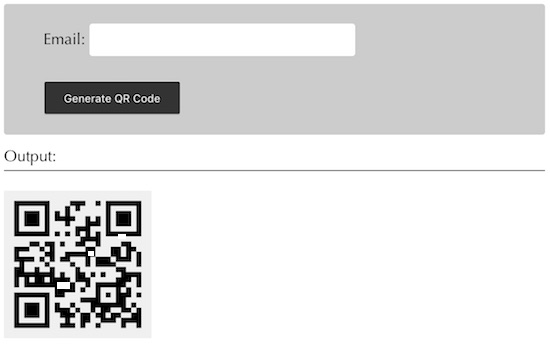# How to Generate QR Code in PHP

The QR code or Quick Response code is a kind of 2D barcode used to store information. Initially, it is designed for the automotive industry but later it is used across all domains for the simplicity of data storing mechanism. In QR code the data storage can be done with the various type of encoding techniques. The stored data are machine-readable and can be interpreted by a reader or using a camera like smartphone apps.There are various PHP libraries available to generate QR code. In this article, we are going to get the email address from the user and store it in the form of QR code. I am using tc-lib-barcode PHP library to generate QR code to store data. I get the user email via a HTML form. On submitting the form I validated the user email input and apply the tc-lib-barcode library function to encode and store the email address data in the form of QR code. This data can be interpreted by any QR code scanner.

## Getting User Data via HTML to Store in QR Code

This page contains a HTML form to get the user email address. On submitting this form, I call the jQuery validation script to check the user input and its format. If the user enters the email address in the correct format then the form will be submitted successfully.

```<form method="post" name="frmQRGenerator" id="frm-qr"
onSubmit="return validate();">
<div class="form-row">
Email: <input type="text" name="email_field" id="email_field"
class="input-field" />
</div>

<div>
<input type="submit" name="generate" class="submit-button"
value="Generate QR Code" />
</div>
</form>

<div id="validation-info"></div>
<script src="jquery-3.2.1.min.js"></script>
<script>
function validate() {
var valid = true;
var emailRegexp = /^((([a-z]|\d|[!#\\$%&'\*\+\-\/=\?\^_`{\|}~]|[\u00A0-\uD7FF\uF900-\uFDCF\uFDF0-\uFFEF])+(\.([a-z]|\d|[!#\\$%&'\*\+\-\/=\?\^_`{\|}~]|[\u00A0-\uD7FF\uF900-\uFDCF\uFDF0-\uFFEF])+)*)|((\x22)((((\x20|\x09)*(\x0d\x0a))?(\x20|\x09)+)?(([\x01-\x08\x0b\x0c\x0e-\x1f\x7f]|\x21|[\x23-\x5b]|[\x5d-\x7e]|[\u00A0-\uD7FF\uF900-\uFDCF\uFDF0-\uFFEF])|(\\([\x01-\x09\x0b\x0c\x0d-\x7f]|[\u00A0-\uD7FF\uF900-\uFDCF\uFDF0-\uFFEF]))))*(((\x20|\x09)*(\x0d\x0a))?(\x20|\x09)+)?(\x22)))@((([a-z]|\d|[\u00A0-\uD7FF\uF900-\uFDCF\uFDF0-\uFFEF])|(([a-z]|\d|[\u00A0-\uD7FF\uF900-\uFDCF\uFDF0-\uFFEF])([a-z]|\d|-|\.|_|~|[\u00A0-\uD7FF\uF900-\uFDCF\uFDF0-\uFFEF])*([a-z]|\d|[\u00A0-\uD7FF\uF900-\uFDCF\uFDF0-\uFFEF])))\.)+(([a-z]|[\u00A0-\uD7FF\uF900-\uFDCF\uFDF0-\uFFEF])|(([a-z]|[\u00A0-\uD7FF\uF900-\uFDCF\uFDF0-\uFFEF])([a-z]|\d|-|\.|_|~|[\u00A0-\uD7FF\uF900-\uFDCF\uFDF0-\uFFEF])*([a-z]|[\u00A0-\uD7FF\uF900-\uFDCF\uFDF0-\uFFEF])))\.?\$/i

\$("#validation-info").hide();
\$("#validation-info").html();
if (\$("#email_field").val() == "") {
\$("#validation-info").show();
\$("#validation-info").html("Email is required.");
valid = false;
} else if (!(emailRegexp.test(\$("#email_field").val()))) {
\$("#validation-info").show();
\$("#validation-info").html("Invalid Email.");
valid = false;
}
return valid;
}
</script>
```

## PHP tc-lib-barcode Code to Generate QR Code

Download the tc-lib-barcode library before executing this example in your PHP environment. Using this library functions I have created the barcode object by sending parameters like the dimensions, the data to be stored in the form of barcode and more.

The barcode instance is used to create the image binaries to store the barcode in a PNG format in a specified target folder. The file path will be used to show the QR output to the user. When you scan this output QR code image, the data stored in this 2D QR code will be interpreted.

```<?php
if (! empty(\$_POST["email_field"])) {
\$barcode = new \Com\Tecnick\Barcode\Barcode();
\$targetPath = "qr-code/";

if (! is_dir(\$targetPath)) {
mkdir(\$targetPath, 0777, true);
}
\$bobj = \$barcode->getBarcodeObj('QRCODE,H', \$_POST["email_field"], - 16, - 16, 'black', array(
- 2,
- 2,
- 2,
- 2
))->setBackgroundColor('#f0f0f0');

\$imageData = \$bobj->getPngData();
\$timestamp = time();

file_put_contents(\$targetPath . \$timestamp . '.png', \$imageData);
?>